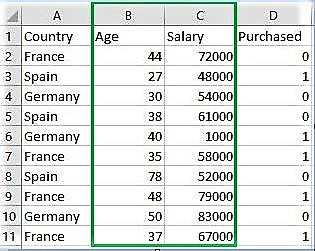Skip to content
Related Articles
ML | Feature Scaling – Part 2
• Difficulty Level : Easy
• Last Updated : 24 Jul, 2019

Feature Scaling is a technique to standardize the independent features present in the data in a fixed range. It is performed during the data pre-processing to handle highly varying magnitudes or values or units. If feature scaling is not done, then a machine learning algorithm tends to weigh greater values, higher and consider smaller values as the lower values, regardless of the unit of the values.

Example: If an algorithm is not using feature scaling method then it can consider the value 3000 meter to be greater than 5 km but that’s actually not true and in this case, the algorithm will give wrong predictions. So, we use Feature Scaling to bring all values to same magnitudes and thus, tackle this issue.

Techniques to perform Feature Scaling
Consider the two most important ones:

• Min-Max Normalization: This technique re-scales a feature or observation value with distribution value between 0 and 1.• Standardization: It is a very effective technique which re-scales a feature value so that it has distribution with 0 mean value and variance equals to 1.Download the dataset:
Go to the link and download Data_for_Feature_Scaling.csvBelow is the Python Code:

 `# Python code explaining How to``# perform Feature Scaling``  ` `""" PART 1``    ``Importing Libraries """``  ` `import` `numpy as np``import` `matplotlib.pyplot as plt``import` `pandas as pd`` ` `# Sklearn library ``from` `sklearn ``import` `preprocessing`` ` `""" PART 2``    ``Importing Data """``  ` `data_set ``=` `pd.read_csv(``'C:\\Users\\dell\\Desktop\\Data_for_Feature_Scaling.csv'``)``data_set.head()`` ` `# here Features - Age and Salary columns ``# are taken using slicing``# to handle values with varying magnitude``x ``=` `data_set.iloc[:, ``1``:``3``].values``print` `(``"\nOriginal data values : \n"``,  x)`` ` ` ` `""" PART 4``    ``Handling the missing values """`` ` `from` `sklearn ``import` `preprocessing`` ` `""" MIN MAX SCALER """`` ` `min_max_scaler ``=` `preprocessing.MinMaxScaler(feature_range ``=``(``0``, ``1``))`` ` `# Scaled feature``x_after_min_max_scaler ``=` `min_max_scaler.fit_transform(x)`` ` `print` `(``"\nAfter min max Scaling : \n"``, x_after_min_max_scaler)`` ` ` ` `""" Standardisation """`` ` `Standardisation ``=` `preprocessing.StandardScaler()`` ` `# Scaled feature``x_after_Standardisation ``=` `Standardisation.fit_transform(x)`` ` `print` `(``"\nAfter Standardisation : \n"``, x_after_Standardisation)`

Output :

```   Country  Age  Salary  Purchased
0   France   44   72000          0
1    Spain   27   48000          1
2  Germany   30   54000          0
3    Spain   38   61000          0
4  Germany   40    1000          1

Original data values :
[[   44 72000]
[   27 48000]
[   30 54000]
[   38 61000]
[   40  1000]
[   35 58000]
[   78 52000]
[   48 79000]
[   50 83000]
[   37 67000]]

After min max Scaling :
[[ 0.33333333  0.86585366]
[ 0.          0.57317073]
[ 0.05882353  0.64634146]
[ 0.21568627  0.73170732]
[ 0.25490196  0.        ]
[ 0.15686275  0.69512195]
[ 1.          0.62195122]
[ 0.41176471  0.95121951]
[ 0.45098039  1.        ]
[ 0.19607843  0.80487805]]

After Standardisation :
[[ 0.09536935  0.66527061]
[-1.15176827 -0.43586695]
[-0.93168516 -0.16058256]
[-0.34479687  0.16058256]
[-0.1980748  -2.59226136]
[-0.56487998  0.02294037]
[ 2.58964459 -0.25234403]
[ 0.38881349  0.98643574]
[ 0.53553557  1.16995867]
[-0.41815791  0.43586695]]
```My Personal Notes arrow_drop_up